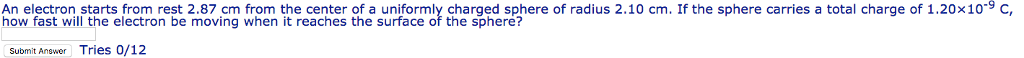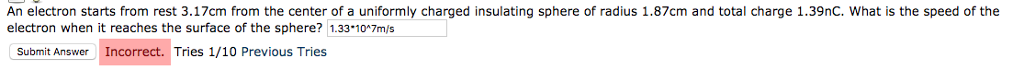# An electron starts from rest 2.87 cm from the center of a uniformly charged sphere of...An electron starts from rest 2.87 cm from the center of a uniformly charged sphere of radius 2.10 cm. If the sphere carries a total charge of 1.20×10-9 C, how fast will the electron be moving when it reaches the surface of the sphere? Submit Answer Tries 0/12

We need at least 10 more requests to produce the answer.

0 / 10 have requested this problem solution

The more requests, the faster the answer.

All students who have requested the answer will be notified once they are available.

#### Earn Coin

Coins can be redeemed for fabulous gifts.

Similar Homework Help Questions
• ### An electron starts from rest 2.96 cm from the center of a uniformly charged sphere of...

An electron starts from rest 2.96 cm from the center of a uniformly charged sphere of radius 2.04 cm. If the sphere carries a total charge of 1.08×10-9 C, how fast will the electron be moving when it reaches the surface of the sphere?

• ### Course Cot. HW 03 (Feb. 05) Electron approaching a charged sphere TimerEvaluate An electron starts from...Course Cot. HW 03 (Feb. 05) Electron approaching a charged sphere TimerEvaluate An electron starts from rest 3.01 cm from the center of a uniformly charged sphere of radius 2.02 cm. If the sphere carries a total charge of 1.12×10-9 C, how fast will the electron be moving when it reaches the surface of the sphere? Tries 0/12

• ### thank you! please use more digits! An electron starts from rest 3.17 cm from the center...thank you! please use more digits! An electron starts from rest 3.17 cm from the center of a uniformly charged insulating sphere of radius 1.87 cm and total charge 1.39 nC. What is the speed of the electron when it reaches the surface of the sphere? 1.33 astir 10^7 m/s Tries 1/10 Previous Tries

• ### An electron starts from red 4.00 an fron, the conter of a uniformly charged sphere of...An electron starts from red 4.00 an fron, the conter of a uniformly charged sphere of radus 2.00 em. " the sphere carnes a total charge of 1.00 x 10, С, how fast will the electron be moving when it reaches the surface of the sphere? m/s

• ### A thin, uniformly charged spherical shell has a potential of 645 V on its surface. Outside...

A thin, uniformly charged spherical shell has a potential of 645 V on its surface. Outside the sphere, at a radial distance of 21.0 cm from this surface, the potential is 325 V. Calculate the radius of the sphere. Determine the total charge on the sphere. What is the electric potential inside the sphere at a radius of 2.0 cm? Calculate the magnitude of the electric field at the surface of the sphere. If an electron starts from rest at...

• ### An electron is released from rest on the axis of a uniform positively charged ring, 0.111m...

An electron is released from rest on the axis of a uniform positively charged ring, 0.111m from the ring's center. If the linear charge density of the ring is +0.104nC/m and the radius of the ring is 0.222m, how fast will the electron be moving when it reaches the center of the ring?

• ### A uniformly charged, thin ring has radius 15.5 cm and total charge +23.3 nC. An electron...

A uniformly charged, thin ring has radius 15.5 cm and total charge +23.3 nC. An electron is placed on the ring’s axis a distance 26.1 cm from the center of the ring and is constrained to stay on the axis of the ring. The electron is then released from rest. Find the speed of the electron when it reaches the center of the ring. (Give your answer in scientific notation using m/s as unit)

• ### Uniformly charged sphere.

22.4 A uniformly charged solid sphere with a total charge of 50 nC and a radius R_1 = 30 cm is surrounded concentrically by a thin spherical shell. The thin shell has a total charge of –17 nC and a radius R_2 = 45 cm. Find the electric field at distances of a)14 cm, b) 37 cm, and c) 52 cm from the center of the sphere.

• ### A uniformly charged thin ring...

A uniformly charged thin ring has radius 15.0 cm and total charge 23.5 nC. An electron is placed on the ring's axis a distance 30.5 cm from the center of the ring andis constrained to stay on the axis of the ring. The electron is then released from rest. Describe the subsequent motion of the electron. Find the speed of the electronwhen it reaches the center of the ring.

• ### An electron starts from rest 31.0 cm from a fixed point charge with Q = -7.50...

An electron starts from rest 31.0 cm from a fixed point charge with Q = -7.50 nC . Part A How fast will the electron be moving when it is very far away?